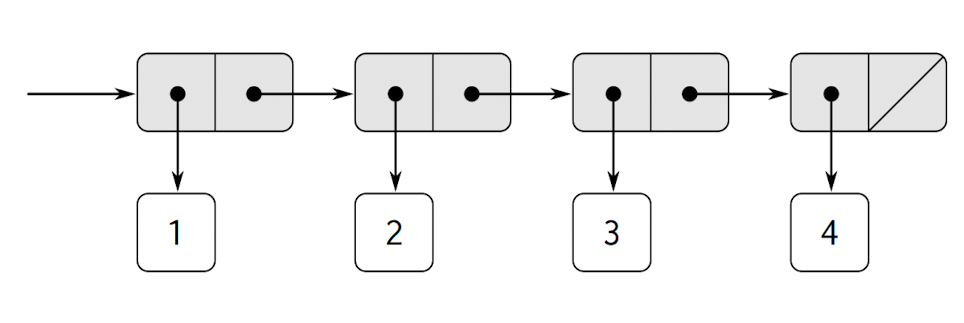﻿ 基于Python实现层次性数据和闭包性质_python_脚本之家
python# 基于Python实现层次性数据和闭包性质

## 绪论## 1.序列的表示```class LinkedList():
def __init__(self, *items) -> None:
"""提供两种初始化方式:序对或多个元素
"""
if isinstance(items, list):
self.pair = items
else:
self.pair = self._construct(*items)

def _construct(self, *items):
"""递归地构造链表
"""
if items == ():
return None
else:
item, *rest = items
return [item, self._construct(*rest)]

def __repr__(self):
"""重写打印函数
"""
return "-->".join(map(str, self._flatten(self.pair)))

def _flatten(self, pair):
"""遍历链表，返回其一维展开
"""
if pair is None:
return []
else:
return [pair] + self._flatten(pair)

@property
"""获取链表头部元素
"""
return self.pair

@property
def rest(self):
"""获取链表头部元素之外的元素，并以链表形式返回
"""
if self.pair is None:
return None
else:

```print(LinkedList(1, 2, 3, 4))
# 1-->2-->3-->4
```

### 表操作

```def list_ref(items, n):
if n == 0:
else:
return list_ref(items.rest, n-1)
print(list_ref(LinkedList(1, 4, 9, 16, 25), 3)) # 16```

`length`过程则用于返回表中的项数：

```def length(items):
if items is None:
return 0
else:
return 1 + length(items.rest)

print(length(LinkedList(1, 3, 5, 7))) # 4
```

```# 以迭代的方式计算lengths(尾递归)
def length(items):
def length_iter(a, count):
if a is None:
return count
else:
return length_iter(a.rest, count + 1)
return length_iter(items, 0)

print(length(LinkedList(1, 3, 5, 7))) # 4
```

```def append(lk_list1, lk_list2):
if lk_list1 is None:
return lk_list2.pair
else:

odds = LinkedList(1, 3, 5, 7)
squares = LinkedList(1, 4, 9, 16, 25)
```

### 对链表的映射

```def scale_list(items, factor):
if items is None:
return None
else:
return [items.head * factor, scale_list(items.rest, factor)]

# 10-->20-->30-->40-->50
```

```def my_map(proc, items):
if items is None:
return None
else:

# 10-->2.5-->11.6-->17
# 1-->4-->9-->16-->25
```

```def scale_list(items, factor):

print(scale_list(LinkedList(1, 2, 3, 4, 5), 10))
# 10-->20-->30-->40-->50
```

`map`是一种很重要的结构，不仅因为它代表了一种公共模式，而且因为它建立起了一种处理表的高层抽象（与今日的Scala何其相似！），在老版本的`scale_list`中，程序的递归结构将人的注意力吸引到对表中元素的逐个处理中。通过`map`定义的`scale_list`抑制了这种细节层面上的情况，强调的是从元素表到结果表的一个缩放变换。这两种定义形式之间的差异，并不在于计算机会执行不同的计算过程（其实不会），而在于我们对同一个过程的不同思考方式。 从作用上看，`map`帮我们建起了一层抽象屏障，将实现表转换过程的实现，与与如何提取表中元素以及组合结果的细节隔离开。

## 2.层次性结构```def count_leaves(tree):
if not tree:
return 0
elif isinstance(tree, int):
return 1
else:
return count_leaves(tree) + count_leaves(tree[1:])
tree = [[1, 2], 3, 4]
print(count_leaves(tree)) # 4
```

### 对树的映射

`map`是处理序列的一种强有力抽象，与此类似，`map`与递归结合也是处理树的一种强有力抽象。类似于`2.2.1`中用`scale_list`过程对序列元素进行缩放，我们也可以设计`scale_tree`过程，该过程以一个因子和一棵叶子为数值的树作为参数，返回一颗具有同样形状的树，该树中的每个数值都乘以了这个因子：

```def scale_tree(tree, factor):
if not tree:
return []
if isinstance(tree, int):
return tree * factor
else:
return [scale_tree(tree, factor)] + scale_tree(tree[1:], factor)

tree = [1, [2, [3, 4], 5], [6, 7]]
print(scale_tree(tree, 10))
# [10, [20, [30, 40], 50], [60, 70]]
```

```def scale_tree(tree, factor):
return list(map(lambda sub_tree: scale_tree(sub_tree, factor)
if isinstance(sub_tree, list)
else sub_tree * factor, tree))
tree = [1, [2, [3, 4], 5], [6, 7]]
print(scale_tree(tree, 10))
# [10, [20, [30, 40], 50], [60, 70]]
```

```def my_map(proc, items):
if items == []:
return []
else:
return [proc(items)] + my_map(proc, items[1:])
```

## 3.序列做为一种约定的界面

```def sum_odd_squares(tree):
if not tree:
return 0
elif isinstance(tree, int):
if tree % 2 == 1:
return tree**2
else:
return 0
else:
return sum_odd_squares(tree) + sum_odd_squares(tree[1:])
```

```def fib(n):
if n == 0:
return 0
elif n == 1:
return 1
else:
return fib(n-1) + fib(n-2)
```

```def even_fibs(n):  # 枚举从0到n的整数
def next(k):
if k > n:
return []
else:
f = fib(k)  # 对每个整数计算其fib
if f % 2 == 0:  # 过滤结果，选出其中偶数
return [f] + next(k + 1)  # 累积结果
else:
return next(k+1)
return next(0)
print(even_fibs(5)) # [0, 2] (即[0 1 1 2 3 5]中的偶数为[0, 2])
```

• 枚举出一棵树的树叶
• 过滤它们，选出其中的奇数
• 对选出的每一个数求平方
• 用+累积起得到的结果

• 枚举从00到nn的整数
• 对每个整数计算相应的Fibonacci数
• 过滤它们，选出其中的偶数
• `connect`累计得到的结果

```def con(x, y):
# y规定为int，x可以为int或list
if isinstance(x, int):
return [x] + [y]
else:
return x + [y]
``````def my_map(proc, sequence):
if not sequence:
return []
else:
return [proc(sequence)] + my_map(proc, sequence[1:])

print(my_map(lambda x: x**2, [1, 2, 3, 4, 5]))
# [1, 4, 9, 16, 25]

def my_filter(predicate, sequence):
if not sequence:
return []
elif predicate(sequence):
return [sequence] + my_filter(predicate, sequence[1:])
else:
return my_filter(predicate, sequence[1:])

print(my_filter(lambda x: x % 2, [1, 2, 3, 4, 5]))
# [1, 3, 5]

# print(list(accumulate([1,2,3])))

def my_reduce(op, sequence):
if sequence[-1] and not sequence[:-1]:
return sequence[-1]
else:
return op(my_reduce(op, sequence[:-1]), sequence[-1])

print(my_reduce(add, [1, 2, 3, 4, 5])) # 15
print(my_reduce(mul, [1, 2, 3, 4, 5])) # 120
print(my_reduce(con, [1, 2, 3, 4, 5])) # [1, 2, 3, 4, 5]
```

```def enumerate_interval(low, high):
if low > high:
return []
else:
return [low] + enumerate_interval(low + 1, high)

print(enumerate_interval(2, 7)) # [2, 3, 4, 5, 6, 7]
```

```# 枚举一棵树所有的树叶：
def enumerate_tree(tree):
if not tree:
return []
elif isinstance(tree, int):
return [tree]
else:
return enumerate_tree(tree) + enumerate_tree(tree[1:])

print(enumerate_tree([1, [2, [3, 4], 5]])) # [1, 2, 3, 4, 5]
```

`sum_odd_squares`的构造方法如下：

```def sum_odd_squares(tree):
map(lambda x: x**2,
filter(lambda x: x % 2,
enumerate_tree(tree))))

print(sum_odd_squares([1, 2, 3, 4, 5])) # 35
```

`even-fibs`的构造方法如下：

```def even_fibs(n):
return reduce(con,
filter(lambda x: not x % 2,
map(fib,
enumerate_interval(0, n))))

print(even_fibs(5)) #[0, 2]
```

```def list_fib_squares(n):
return reduce(con,
map(lambda x: x**2,
map(fib,
enumerate_interval(0, n))))

print(list_fib_squares(5)) # [0, 1, 1, 4, 9, 25]
```

```def product_of_squares_of_odd_elements_sequence(sequence):
return reduce(mul,
map(lambda x: x**2,
filter(lambda x: x % 2, sequence)))

print(product_of_squares_of_odd_elements_sequence([1, 2, 3, 4, 5])) # [0, 1, 1, 4, 9, 25]
```

```def salary_of_hightest_paid_programmer(records):
return reduce(max,
map(salary,
filter(is_programmer, records)))
```